TitleCollege Algebra
Tutorial 41: Practice Test on Tutorials 34 - 40Learning Objectives

 After completing this tutorial, you should be able to: Take a test on topics covered in tutorials 34 - 40 in this website.

 Special Notes about Tutorial 41: I can not guarantee you will pass your test after going though any of the tutorials in this website or this practice test. However, it will definitely help you to better understand the topics covered better. Disclaimer: WTAMU and Kim Seward are not responsible for how a student does on any test or any class for any reason including not being able to access the website due to any technology problems.  There are no videos on this page.Introduction

It is important to note that, chances, are I'm not your math instructor.  You need to check with your math teacher as to things like when your next math test is and what it covers.  It may cover more material on the test than what is in this practice test.  Just note that there are other practice tests at this website. So, after finding out what is on your test (if you have one) do the practice test(s) problems that go with the test you are preparing for.  If you are not in a class or are not having a test soon, this practice test is still good practice to go through and check to make sure you are understanding this material before moving on - kind of like a spot check. The material on this practice test goes with Tutorial 34: Graphs of Quadratic Functions, Tutorial 35: Graphs of Polynomial Functions, Tutorial 36:  Long Division, Tutorial 37: Synthetic Division and the Remainder and Factor Theorems, Tutorial 38: Zeros of Polynomial Functions, Part I, Tutorial 39: Zeros of Polynomial Functions, Part II, and Tutorial 40: Graphs of Rational Functions.

Also note that your teacher may word the problems on their test a little differently, may have some different kinds of problems, or may have a different number of problems than what is in this practice test.  Again, since I'm probably not your math instructor, I don't know exactly how your teacher will set up your math test.  Just note that these problems will help you build an understanding of the concepts presented and the terms used in math problems.  If you have an understanding of the problems instead of just memorizing them, then you should do fine on these concepts, no matter how the test is set up.

 Steps to Studying for a Math Test
1. Work through problems.  If you are in a class, you should have done this on completion of any homework you have done.  For anyone,  you can accomplish this by doing the practice problems found in each tutorial.

2. Check work on problems.  The practice problems in each tutorial have links to the answers to them so you can instantly check how you are doing.  Also, in most math books, the odd answers are found in the back of the book.

3. Review concepts.  Whether you got the problems right or wrong, make sure you review over them.  If you did get a problem wrong, make sure you either review that concept in it's respective tutorial or ask your math teacher about it.  If you don't ask about a problem before a test,  you are going to kick yourself when it comes up on the test.

4. Work through problems as if you were taking the test - no notes, book, webpages, etc.  This practice test is a perfect way to do that. After taking this practice test, check your answers by clicking on the link to the answer key found at the bottom of the practice test (before the 'need extra help on these topics' section)

 During the Test

It is to your benefit to show as much of the work as possible on the problems that have several steps involved.

Make sure that you read the directions carefully,  you wouldn't believe how many points get taken off  math tests for people not following directions.

Pace yourself.  You do not have to be the first one done to do well on the test.  Do not panic if there is still time left to take the test and others are turing it in.  Sometimes that means they do not know the material and left some of the answers blank.  Do not worry about anyone else but yourself.

Don't rush through a problem.  Another thing that math teachers take points off for are careless mistakes made by people that rush through a problem.  When those students get their tests back, they bonk themselves on the head at some of the things that got counted wrong,  things that they knew how to do.

Remember to breathe!!!!  I know some of you are scared to death at the thought of having to take a math test of any kind.  For you guys, try to relax and don't forget to breathe.  (Even if you aren't scared to take a math test,  it is probably a good idea to remember to breathe,  I wouldn't want you to pass out during the test). If it feels like your brain has left the building during your test, just close your eyes and breathe in and out and in and out and your brain will return.

Good luck on your test.  If you are taking a math test soon, don't panic, you are going to do great!!!Practice Test

 Problems 1a - 1b:  Find the coordinates of the vertex of the given quadratic function.  Without graphing, determine if the vertex is the maximum or minimum point of the quadratic function.

 1a.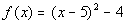1b.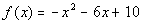Problems 2a - 2b:  Use the vertex and the intercepts to sketch the graph of the given quadratic function.  Find the equation for this function’s axis of symmetry.

 2a.2b.Problem 3a:  Given the polynomial function a) use the Leading Coefficient Test to determine the graph’s end behavior, b) find the x-intercepts (or zeros) and state whether the graph crosses the x-axis or touches the x-axis and turns around at each x-intercept, c) find the y-intercept, d) determine the symmetry of the graph, e) indicate the maximum possible turning points, and f) graph.

 3a.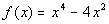Problem 4a:  Divide using long division.

 4a.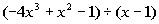Problem 5a: Divide using synthetic division.

 5a.Problem 6a:  Given the function f(x), use the Remainder Theorem to find f(-2).

 6a.Problem 7a:  Solve the given equation given that 2/3 is a zero (or root) of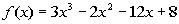.

 7a.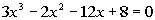Problems 8a - 8b:  List all of the possible zeros or roots, use Descartes’s Rule of Signs to possible narrow it down, use synthetic division to test the possible zeros or roots and find an actual zero or root, and use the actual zero to find all zeros or the actual root to find all solutions to the given polynomial function or equation to solve.

 8a.8b.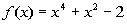Problem 9a:  Show that all real roots of the given equation lie between -2 and 2.

 9a.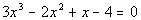Problem 10a:  Show that the given polynomial has a real zero between the given integers.  Use the Intermediate Value theorem to find an approximation for this zero to the nearest tenth.

 10a.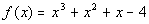;  between 1 and 2.

 Problem 11a:  Use the given root to find all of the roots of the given polynomial equation.

 11a.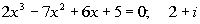Problem 12a:  Factor the given polynomial function  a) as the product of factors that are irreducible over rational numbers, b) as the product of factors that are irreducible over real numbers, and c) in completely factored form involving complex nonreal numbers.

 12a.Problem 13a:  Find an nth degree polynomial function with the given conditions.

 13a. n = 3;  -3i and 2 are zeros and f(1) = -20

 Problem 14a:  Sketch the graph of the rational function.

 14a.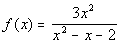Now you are ready to check the answers to your practice test: Answer key to practice testNeed Extra Help on these Topics?

The following are web pages that can assist you in the topics that were covered on this page:

Go to Get Help Outside the Classroom found in Tutorial 1: How to Succeed in a Math Class for some more suggestions.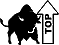Last revised on March 20, 2011 by Kim Seward.
All contents copyright (C) 2002 - 2011, WTAMU and Kim Seward. All rights reserved.